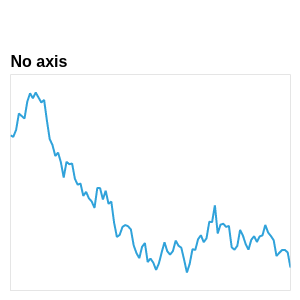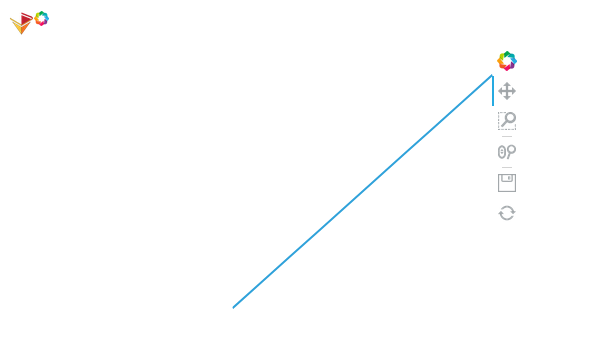# Remove frame around plot

Hello everyone,

in any graph there is a subtle frame around the figure even if the axis of the plot is removed. Examples of this can be seen throughout the holoviews documentation, for instance here in section ‘Axis Position’:
https://holoviews.org/user_guide/Customizing_Plots.html
For convinience, below is the image I’m referring to:I would like to remove this frame. How would I go about doing that?
Any ideas would be appreciated.

I haven’t got the opportunity to try currently on the holiday bops but you can see if this bokeh option is avail to use maybe

1 Like

Thanks for the quick reply, that looks promising!
But I’m not quite sure yet how to integrate this code into the setup for my graph.
Below I’ve created a simplified graph with two nodes and one connecting edge.
I’ve removed the x-and y-axis already and now only need to get rid of the surrounding frame.

Could you please tell me what code line(s) I would need to add to my example?

``````import numpy as np
import holoviews as hv
hv.extension('bokeh')

# function that creates a graph with two nodes and one connecting edge and sets its edge color to the given
# function parameters
def hv_graph(edge_color):
node_indices = np.arange(2, dtype=np.int32)
source = np.zeros(2, dtype=np.int32)
target = node_indices
graph = hv.Graph(((source, target),)).opts(edge_color=edge_color)
graph.opts(xaxis=None,yaxis=None, title="")
return graph

def main():
# create the dynamic map
dmap = hv.DynamicMap(hv_graph, kdims=["edge_color"])
dmap = dmap.redim.values(edge_color=["green", "black"])
# save the dynamic map
hv.save(dmap, 'dmap.html', backend='bokeh')
return

if __name__ == '__main__':
main()
``````

The way to integrate it is to use plot hooks.

Thanks, that looks like it should work, I’ll try that.

1 Like

Hi again,

I’ve tried to make a plot hook out of the blog entry provided by carl, but it doesn’t seem to work. I’m not too familiar with plot hooks or bokeh, so I might have made a mistake.

Could you give me some pointers on how to make the necessary plot hook?

This is my plot hook so far:

``````def hook(plot, element):
plot.handles["border_fill_color"]=None
``````

This does the trick``````import holoviews as hv
hv.extension("bokeh")

def hide_hook(plot, element):
plot.handles["xaxis"].visible = False
plot.handles["yaxis"].visible = False
# plot.handles["xgrid"].visible = False
# plot.handles["ygrid"].visible = False
plot.handles["plot"].border_fill_color = None
plot.handles["plot"].background_fill_color = None
plot.handles["plot"].outline_line_color = None

hv.Curve(range(10)).opts(hooks=[hide_hook])'
``````2 Likes

Thanks a lot, that works great